# Mesh Analysis

## What is Mesh Analysis?

Mesh analysis is defined as

The method in which the current flowing through a planar circuit is calculated.

A planar circuit is defined as the circuits that are drawn on the plane surface in which there are no wires crossing each other. Therefore, a mesh analysis can also be known as loop analysis or mesh-current method.

### What is Branch?

A branch is defined as the path that connects two nodes such that it contains a circuit element. If the branch belongs to only one mesh, then the branch current and the mesh current will be equal to each other.

## Procedure of Mesh Analysis

The following steps are to be followed while solving the given electrical network using mesh analysis:

Step 1:

To identify the meshes and label these mesh currents in either clockwise or counterclockwise direction.

Step 2:

To observe the amount of current that flows through each element in terms of mesh current.

Step 3:

Writing the mesh equations to all meshes using Kirchhoff’s voltage law and then Ohm’s law.

Step 4:

The mesh currents are obtained by following Step 3 in which the mesh equations are solved.

Hence, for a given electrical circuit the current flowing through any element and the voltage across any element can be determined using the node voltages.

#### Recommended Video:### Mesh Current Diagram

The below diagram is a circuit with mesh currents labelled as I1, I2, and I3 and the arrows represent the direction of the mesh current.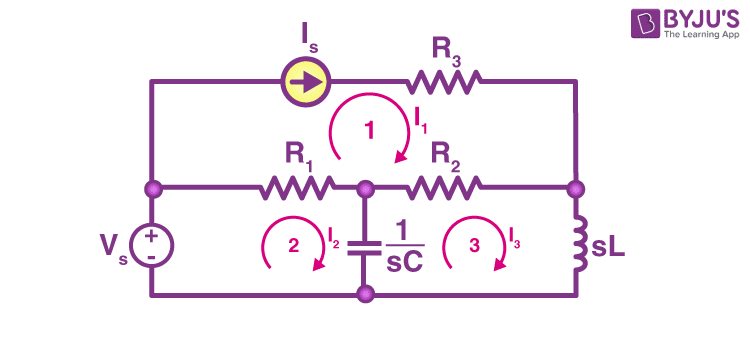## What is Super Mesh Analysis?

Super mesh analysis is used for solving huge and complex circuits in which two meshes share a common component as a source of current.

## Example of Mesh Analysis

Example 1:

In the given circuit 90v is the battery value, 5A is the current source and the three resistors are 9 ohms, 6 ohms, and 8 ohms. Using mesh analysis, determine the current across each resistor and potential difference.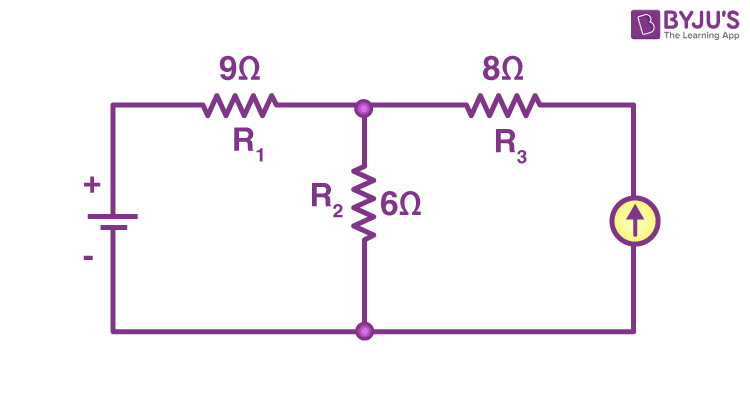Solution:

Let’s first determine the ground as shown in the figure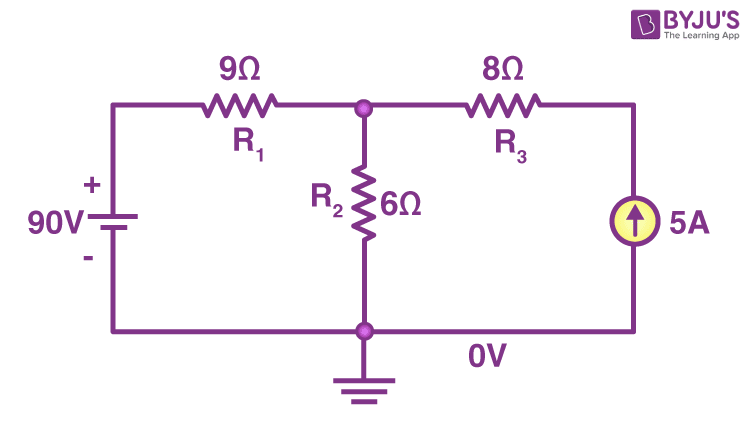Let the potential be VA, VB, and VC as shown in the figure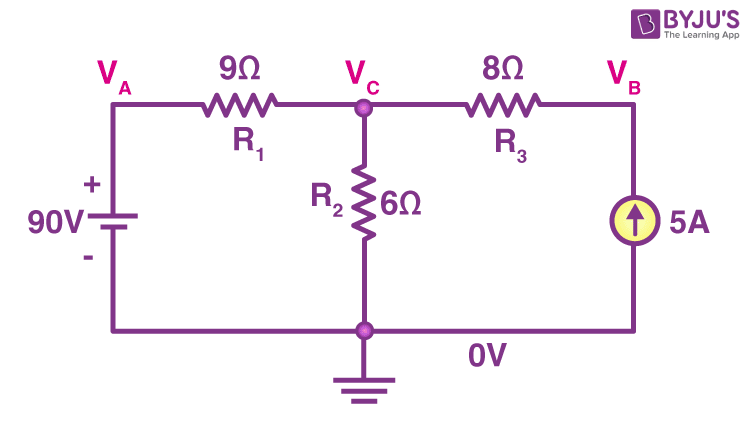Let I1 and I2 be the currents flowing through the two loops in the clockwise direction as shown in the figure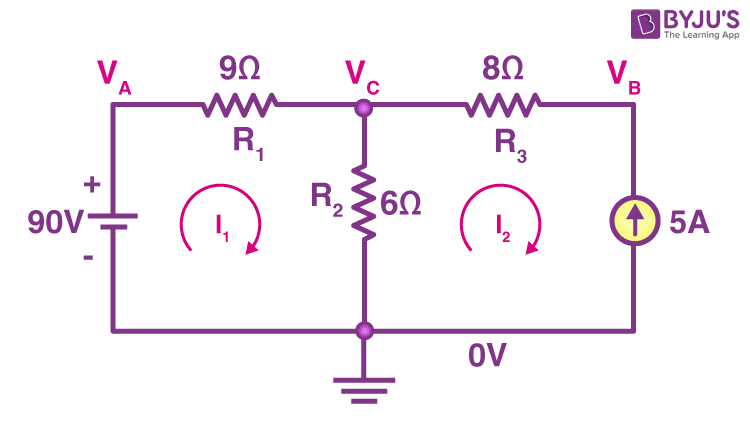Therefore,

+VB – V1 – V2 = 0

90 – I1R1 – R2(I1 – I2) = 0

90 – 9I1 – 6(I1 – I2) = 0

-15I1 + 6I2 = -90

5I1 – 2I2 = 30 (this is obtained by dividing the equation with -3)

Substituting I2 as -5 since the direction of I2 is opposite to the actual direction of current

Therefore,

I1 = 4A

So, through R1, 4A current is flowing and through R3, 5A current is flowing.

Now the potential difference at VA = 90V

At VB, the potential difference is V2 = I2 – R2

Therefore, VB = 54V

At VC, the potential difference is V3 = I3 – R3

VC – 54 = 40

VC = 94V

## Frequently Asked Questions – FAQs

### What is the difference between loop and mesh?

The difference between loop and mesh is that a loop is a closed path in a circuit in which none of the nodes repeat more than once. While a mesh is a closed path in a circuit in which no other paths are present.

### What are the limitations of mesh analysis?

The following are the disadvantages of mesh analysis:

• Mesh analysis is useful only when the circuit is planar.
• As the number of meshes increases, the number of equations increases, which makes it inconvenient for solving.

### Which Kirchhoff’s law is used in mesh analysis?

Kirchhoff’s voltage law is used in mesh analysis.

### What is the difference between mesh and nodal analysis?

The difference between mesh and nodal analysis is that nodal analysis is an application of Kirchhoff’s current law, which is used for calculating the voltages at each node in an equation. While mesh analysis is an application of Kirchhoff’s voltage law which is used for calculating the current.

### What is a dependent source?

A dependent source in an electrical network is defined as either a voltage source or a current source whose value is dependent on the voltage or current in the network. Voltage-controlled voltage source, voltage-controlled current source, current-controlled current source, and current-controlled voltage source are the classification of the dependent sources.